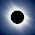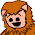## Saturday, May 18, 2013

### How could Scala do a merge sort?

Merge sort is a classical "divide and conquer" sorting algorithm. You should have to never write one because you'd be silly to do that when a standard library class already will already do it for you. But, it is useful to demonstrate a few characteristics of programming techniques in Scala. Firstly a quick recap on the merge sort. It is a divide and conquer algorithm. A list of elements is split up into smaller and smaller lists. When a list has one element it is considered sorted. It is then merged with the list beside it. When there are no more lists to merged the original data set is considered sorted. Now let's take a look how to do that using an imperative approach in Java.
```public void sort(int[] values) {
int[] numbers = values;
int[] auxillaryNumbers = new int[values.length];
mergesort(numbers, auxillaryNumbers, 0, values.length - 1);
}

private void mergesort(int [] numbers, int [] auxillaryNumbers, int low, int high) {
// Check if low is smaller then high, if not then the array is sorted
if (low < high) {
// Get the index of the element which is in the middle
int middle = low + (high - low) / 2;

// Sort the left side of the array
mergesort(numbers, auxillaryNumbers, low, middle);
// Sort the right side of the array
mergesort(numbers, auxillaryNumbers, middle + 1, high);

// Combine them both
// Alex: the first time we hit this when there is min difference between high and low.
merge(numbers, auxillaryNumbers, low, middle, high);
}
}

/**
* Merges a[low .. middle] with a[middle..high].
* This method assumes a[low .. middle] and a[middle..high] are sorted. It returns
* a[low..high] as an sorted array.
*/
private void merge(int [] a, int[] aux, int low, int middle, int high) {
// Copy both parts into the aux array
for (int k = low; k <= high; k++) {
aux[k] = a[k];
}

int i = low, j = middle + 1;
for (int k = low; k <= high; k++) {
if (i > middle)                      a[k] = aux[j++];
else if (j > high)                   a[k] = aux[i++];
else if (aux[j] < aux[i])            a[k] = aux[j++];
else                                 a[k] = aux[i++];
}
}

public static void main(String args[]){
...
ms.sort(new int[] {5, 3, 1, 17, 2, 8, 19, 11});
...
}

}
```
Discussion...
1. An auxillary array is used to achieve the sort. Elements to be sorted are copied into it and then once sorted copied back. It is important this array is only created once otherwise there can be a performance hit from extensive array created. The merge method does not have to create an auxiliary array however since it changes an object it means the merge method has side effects.
2. Merge sort big(O) performance is N log N.
Now let's have a go at a Scala solution.
```  def mergeSort(xs: List[Int]): List[Int] = {
val n = xs.length / 2
if (n == 0) xs
else {
def merge(xs: List[Int], ys: List[Int]): List[Int] =
(xs, ys) match {
case(Nil, ys) => ys
case(xs, Nil) => xs
case(x :: xs1, y :: ys1) =>
if (x < y) x::merge(xs1, ys)
else y :: merge(xs, ys1)
}
val (left, right) = xs splitAt(n)
merge(mergeSort(left), mergeSort(right))
}
}
```
Key discussion points:
1. It is the same divide and conquer idea.
2. The splitAt function is used to divide up the data up each time into a tuple. For every recursion this will new a new tuple.
3. The local function merge is then used to perform the merging. Local functions are a useful feature as they help promote encapsulation and prevent code bloat.
4. Neiher the mergeSort() or merge() functions have any side effects. They don't change any object. They create (and throw away) objects.
5. Because the data is not been passed across iterations of the merging, there is no need to pass beginning and ending pointers which can get very buggy.
6. This merge recursion uses pattern matching to great effect here. Not only is there matching for data lists but when a match happens the data lists are assigned to variables:
• `x` meaning the top element in the left list
• `xs1` the rest of the left list
• `y` meaning the top element in the right list
• `ys1` meaning the rest of the data in the right list
This makes it very easy to compare the top elements and to pass around the rest of the date to compare. Would the iterative approach be possible in Java? Of course. But it would be much more complex. You don't have any pattern matching and you don't get a nudge to declare objects as immutable as Scala does with making you make something val or var. In Java, it would always be easier to read the code for this problem if it was done in an imperative style where objects are being changed across iterations of a loop. But Scala a functional recursive approach can be quite neat. So here we see an example of how Scala makes it easier to achieve good, clean, concise recursion and a make a functional approach much more possible.

1.Great post Alex. Looking forward to See more of this type. Though I am not a great Scala fan, but I like to take what best Scala offers.

Javin
http://javarevisited.blogspot.com/

2.But Scala approach is much slower, cause there is a need to produce a lot of intermediate objects, while Java's version use only one auxilary array.

1.Not only that, but the "merge" step can't employ tail recursion. I really don't think this is the right way to approach the problem.

2.@Pointy -- No, you can very easily make merge tail recursive. You just add an extra argument, which is strict in scala, and build it there. There's an example in my post:

http://ericwspringer.blogspot.com/2013/05/sorry-but-no-even-merge-sort-sucks-in.html

but you can just as easily do it with cons'ing

3.@Eric Springer: https://gist.github.com/ramn/5692237 slightly modified, I put lists back into it for nicer pattern matching. This runs in around 280 ms for me. The version you have takes minutes! Be sure to prepend to lists, not append since prepend is a constant time operation.